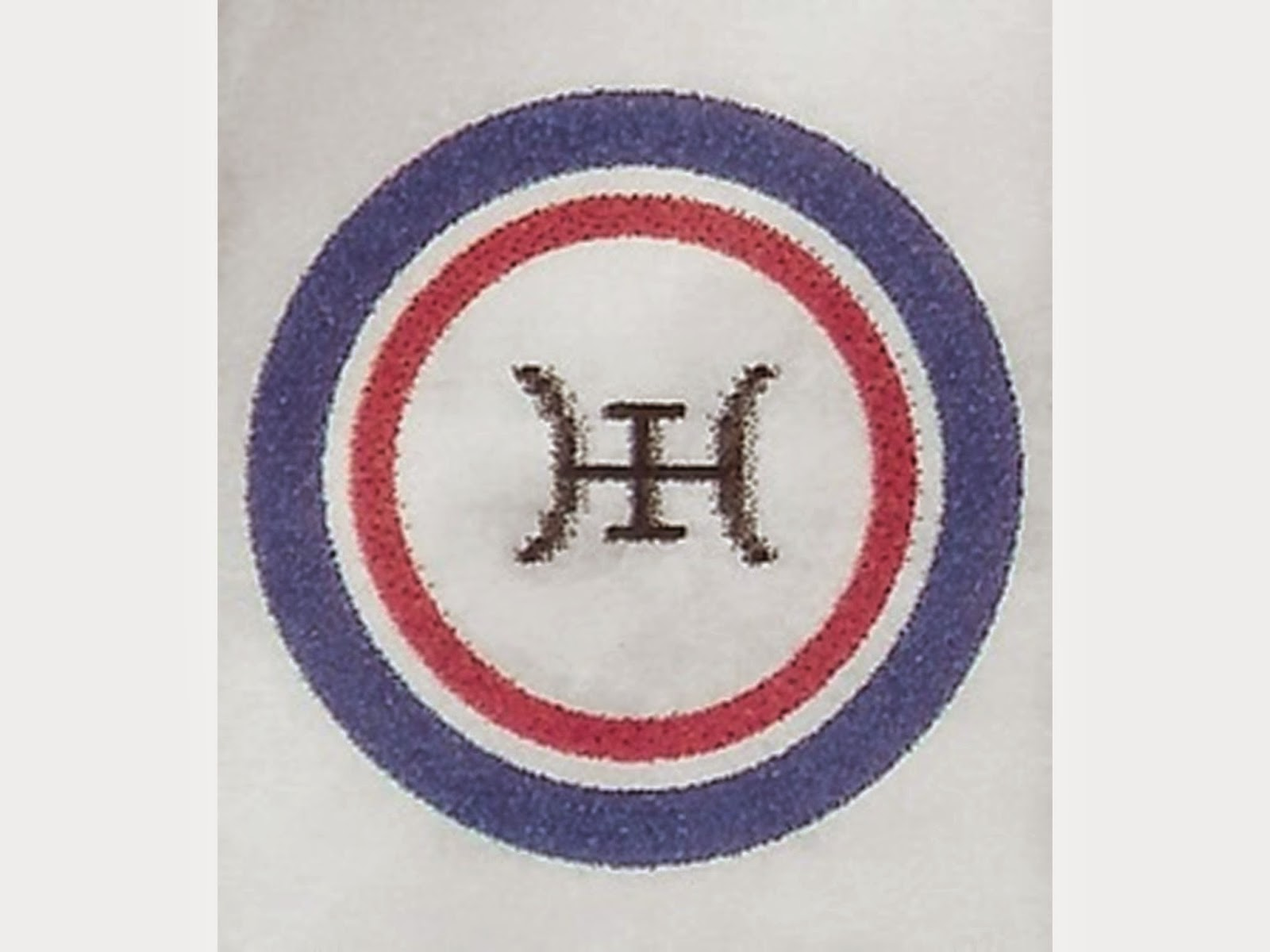## Featured Post

### First Interview with Karl Seldon, F.E.D. Co-Founder & Generalized-Equity Relation-of-Production Discoverer## Friday, May 11, 2018

### New Internet Dialogue Opens on the F.E.D. ‘Dialectical Mathematics’.

New Internet Dialogue Opens on the F.E.D. Dialectical Mathematics.

A reddit participant recently posted, and commented upon, an F.E.D. dialectogram, on one of our conjectures as to the opening triad of our version of the dialectic of Nature, at --

The dialectogramthere posted is a variant of the following --

This posters comments included the following --

“This is true art.  I am actually in awe of how much effort this person has put into this stuff.”

I responded, on behalf of F.E.D., as follows --

“The 9 axioms, grounding the arithmetic/algebra for dialectics applied in the diagram posted above, are stated, within the www.dialectics.org web site, at --

“That these 9 axioms incorporate a version of the first four, “first-order logic” axioms of the “[Dedekind/]Peano Postulates” for the system of arithmetic of the “Natural” Numbers, makes this arithmetic/algebra for dialectic a “non-standard model” of the Natural Numbers.  Such were foretold by the co-application, at the level of “first order” logic, of the Gödel Completeness and Incompleteness Theorems -- two of the deepest theorems of modern “mathematical logic”. An intuitive, dialectical derivation of the system of these “dialectical meta-Natural meta-numbers” from that of the standard “Natural Numbers” is available, within the www.dialectics.org web site, via --

“That these 9 axioms suffice to deductively derive, as a theorem, by a simple direct proof, a STRONG CONTRARY to what Boole called his “fundamental law of thought”, or “law of duality”, which is the core “axiom” of original Boolean algebra, makes this arithmetic/algebra of dialectic a “contra-Boolean algebra” -- an algebraic dialectical logic.  This simple direct proof is presented, within the www.dialectics.org web site, here --

“The resulting dialectical logic is a “supplementary opposite” to Boole’s algebra for formal logic, one that “aufheben”-contains/conserves Boole’s “fundamental law”, but which also “aufheben”-elevates and “aufheben”-negates Boole’s fundamental law for formal logic.  This new, “contra-Boolean” algebra expands Boole’s “law of [EXO-]duality” into a more universal, more general “law of ENDO-duality”.”

“The www.dialectics.org site also recognizes a third axioms-system of dialectical- algebraic logic, one which reconciles the opposition between the Boolean and “contra-Boolean” algebras, in[to] a single ‘uni-system’ of dialectical algebra, incorporating and integrating both.”

“For more about this third system of algebraic dialectical logic, and about the entire, systematic “dialectic/systems-progression of the systems of algebraic [dialectical] logic” -- of “algebras for [dialectical] logic(s)” -- see: